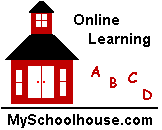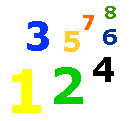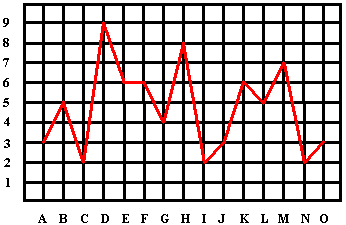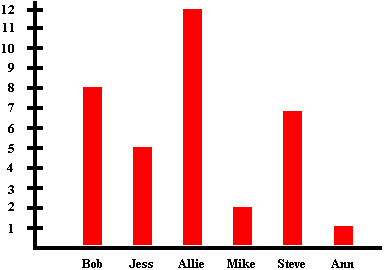# Graphs

Graph Lesson - Line Graphs - Bar Graphs - Elementary Math Lessons & Tests - My Schoolhouse - Online Learning

A graph is a way to show information in a diagram.  Learn to read a line graph and a bar graph.

Look at the examples below.Line Graph

In the line graph above the letter A is shown as 3, the letter D is 9, and the letter M is 7.Bar Graph

In the bar graph above Apples are shown as 10, Pears are shown as 12, and Kiwi are shown as 3.

Read the line graph and bar graph below.How many A's?

How many C's?

How many F's?

How many H's?

How many I's?

How many B's

How many D's?

How many E's?

How many G's

How many J's?

How many N's?How many Bobs?

How many Allies?

How many Jesses?

How many Mikes?

How many Anns?

How many Steves?

 Thank You for using My Schoolhouse